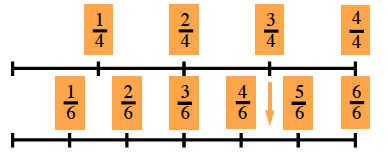Home > CC2MN > Chapter cc23 > Lesson cc23.3.1 > Problem3-94

3-94.

Use any method to divide. Homework Help ✎

1. $\frac{3}{4}\div\frac{1}{6}$

Here is an example of a linear model.1. $\frac { 5 } { 12 } \div ( - \frac { 1 } { 6 } )$

The common denominator is $12$.

$\frac{5}{12}\div\left(-\frac{2}{12}\right)=5\div-2=-\frac{5}{2}=-2\frac{1}{2}$

1. $- \frac { 2 } { 3 } \div \frac { 1 } { 2 }$

What Giant One should you multiply by?

1. $- 2 \frac { 2 } { 3 } \div ( - \frac { 1 } { 2 } )$

Use one of the methods shown in parts (a), (b) or (c).

$\frac{16}{3}=5\frac{1}{3}$